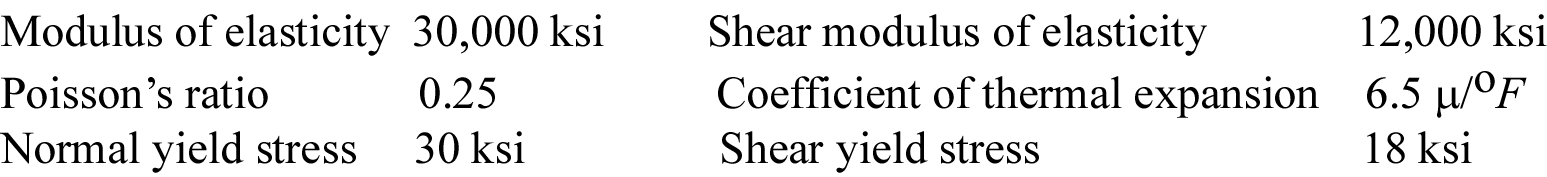# 5.1 – INELASTIC STRUCTURAL BEHAVIOR

For each of the questions below, choose the best answer.Name Email
1.

The axial stress in the circular rod shown in Figure (a) for a temperature increase of 100oF is

2.

The increase of diameter of a circular rod in Figure (a) for a temperature increase of 100oF is

3.

A point in plane strain has the following stress component: σxx= 15 ksi (T) . For a temperature increase of 100oF, the strain εyy is

4.

Each of the two bars in the structure shown in Figure (b) have lengths of 10 inch and cross sectional area of 0.16 inch2. The collapse load is

5.The circular shaft shown in Figure (c) is made from elastic-perfectly plastic material. Due to the action of the torque the section at B was seen to rotate by 0.15 radians. The depth of plastic zone in AB is

6.

The shaft shown in Figure (c) is made from elastic-perfectly plastic material. The depth of plastic zone in AB was found to be 0.35 inch. The maximum torsional shear strain is

7.

The shaft shown in Figure (c) is made from elastic-perfectly plastic material. The value of applied torque when the cross section is fully plastic is

8.

The beam cross section shown in Figure (d) is made from elastic-perfectly plastic material. The magnitude of collapse moment is

9.

The beam cross section shown in Figure (d) is made from elastic-perfectly plastic material. The depth of plastic zone is 0.5 inch. The maximum bending normal strain is

10.

The beam cross section shown in Figure (e) is made from elastic-perfectly plastic material. At collapse moment, the location of the neutral axis from the bottom of the beam is